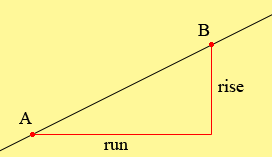SEARCH HOMEMath Central Quandaries & QueriesQuestion from Mak, a student: what is the ratio of the rise to run?Hi Mak

Take two different points A and B on a line as in the diagram below.The rise is the difference between the -coordinates of the two points and the run is the difference between their x-coordinates. The ratio of the rise to the run is called the slope of the line. This is often designated by the letter m so

the slope of the line = m = rise/run.

PennyMath Central is supported by the University of Regina and The Pacific Institute for the Mathematical Sciences.